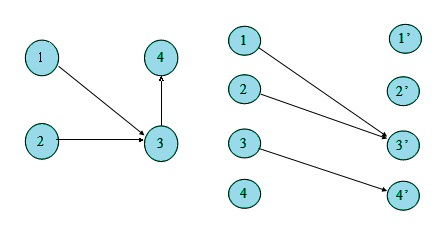# DAG最小路径覆盖模型

## 2.如何求最小路径覆盖？## solution：如果客户u和客户v能用一辆出租车搞定，那么就连一条边。然后跑匈牙利算法。

#include <vector>
#include <cstdio>
#include <iostream>
#include <cstring>
#include <algorithm>
using namespace std;

const int N = 505;

vector<int> G[N];

int line[N], n;
bool S[N], T[N];

bool find(int x){
S[x]=true;
for ( int i=0; i<G[x].size(); i++ ){
int v=G[x][i];
if( !T[v] ){
T[v]=1;
if( line[v]==0 || find( line[v] ) ){
line[v]=x;
return true;
}
}
}
return false;
}

int HUN(){
int ans=0;
memset(line,0,sizeof(line));
for ( int i=1; i<=n; i++ ){
memset(S,0,sizeof(S));
memset(T,0,sizeof(T));
if( find(i) ) ans++;
}
return n-ans;
}

struct Point{
int t, tE;
int xS, yS, xE, yE;
}P[N];

int get_t( Point x, Point y ){
return abs( y.yE-x.yS ) + abs( y.xE-x.xS );
}
int get_tP( Point x ){
return abs( x.yE-x.yS ) + abs( x.xE-x.xS );
}
int x=0; char ch=getchar();
while( !isdigit(ch) ) {
ch=getchar();
}
while( isdigit(ch) ) {
x=x*10+ch-'0';
ch=getchar();
}
return x;
}

int main(){
while( tt-- ){
scanf("%d", &n);
for ( int i=1; i<=n; i++ ) G[i].clear();
for ( int i=1; i<=n; i++ ) {
P[i].tE=P[i].t+get_tP( P[i] );
}
for ( int i=1; i<=n; i++ )
for ( int j=1; j<=n; j++ )
if( i!=j && P[j].t-P[i].tE > get_t( P[j], P[i] ) ) G[i].push_back(j);
printf("%d\n", HUN() );
}
}



08-244643

#### 最小路径覆盖

01-29288

#### DAG最小路径点覆盖05-071937

#### 有向无环图（DAG）的最小路径覆盖

09-03304

#### UVA11419[SAM I AM] 二分图最小覆盖模型

10-09248

#### UVAlive 3126（最小路径覆盖）

03-30263

#### UVAlive3126 Taxi Cab Scheme（DAG的最小路径覆盖）

04-1261

#### UVALive - 3126 Taxi Cab Scheme(二分图最小路径覆盖)

08-22148

#### uvalive3126最小路径覆盖

10-12369

#### UVALive 3126 出租车（DAG的最小不相交路径覆盖）©️2020 CSDN 皮肤主题: 编程工作室 设计师: CSDN官方博客点击重新获取扫码支付1.余额是钱包充值的虚拟货币，按照1:1的比例进行支付金额的抵扣。
2.余额无法直接购买下载，可以购买VIP、C币套餐、付费专栏及课程。余额充值